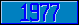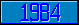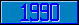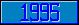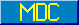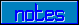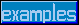## \$%COLLATE

### M[UMPS] by Example

```
16-Feb–1996, 16:54:35

Routine Save for Library function %COLLATE^STRING

;

; The code below was approved in document X11/1996–74

;

COLLATE(A,CHARMOD) NEW X

SET X=""

IF \$GET(CHARMOD)'="" DO

. IF \$EXTRACT(CHARMOD,1)="^" DO

. . SET X=\$EXTRACT(CHARMOD,2,\$LENGTH(CHARMOD))

. . IF X'="" SET X=\$GET(^\$GLOBAL(X,"COLLATE"))

. . QUIT

. IF X="" SET X=\$GET(^\$CHARACTER(CHARMOD,"COLLATE"))

. QUIT

IF X="" SET X=^\$JOB(\$JOB,"COLLATE")

SET X=@(X_"("_X_")")

QUIT x

; ===

;

;

```